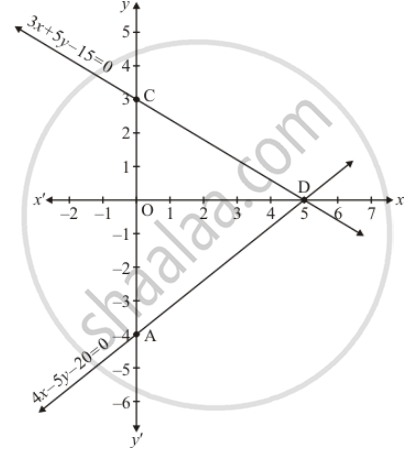Share

# Solve the Following System of Linear Equations Graphically 4x − 5y − 20 = 0 3x + 5y − 15 = 0 Determine the Vertices of the Triangle Formed by the Lines Representing the Above Equation and The Y-axis. - CBSE Class 10 - Mathematics

ConceptGraphical Method of Solution of a Pair of Linear Equations

#### Question

Solve the following system of linear equations graphically

4x − 5y − 20 = 0
3x + 5y − 15 = 0

Determine the vertices of the triangle formed by the lines representing the above equation and the y-axis.

#### Solution

The given equations are:

4x − 5y − 20 = 0 ........(i)

3x + 5y − 15 = 0 ..........(ii)

Putting x = 0 in equation (i) we get:

=> 4 xx 0 - 5y = 20

=> y = -4

x = 0, y - 4

Putting y = 0 in eqaution (i) we get

=> 4x - 5 xx 0 = 20

=> x = 5

x = 5, y = 0

Use the following table to draw the graph.

 x 0 5 y -4 0

Draw the graph by plotting the two points from table3x + 5y = 15 .....(ii)

Putting x = 0  in eqaution (ii) we get

=> 3 xx 0 + 5y = 15

=> x = 5

x = 5, y = 0

Use the following table to draw the graph.

 x 0 5 y 3 0

Draw the graph by plotting the two points from table.

The three vertices of the triangle are A(0, -4), B(5,0), and C(0,3)

Hence the solution of the equation is x = 5 and y = 0

Is there an error in this question or solution?

#### APPEARS IN

Solution Solve the Following System of Linear Equations Graphically 4x − 5y − 20 = 0 3x + 5y − 15 = 0 Determine the Vertices of the Triangle Formed by the Lines Representing the Above Equation and The Y-axis. Concept: Graphical Method of Solution of a Pair of Linear Equations.
S